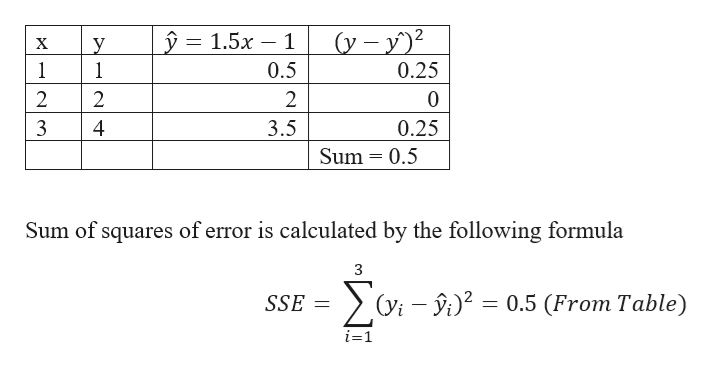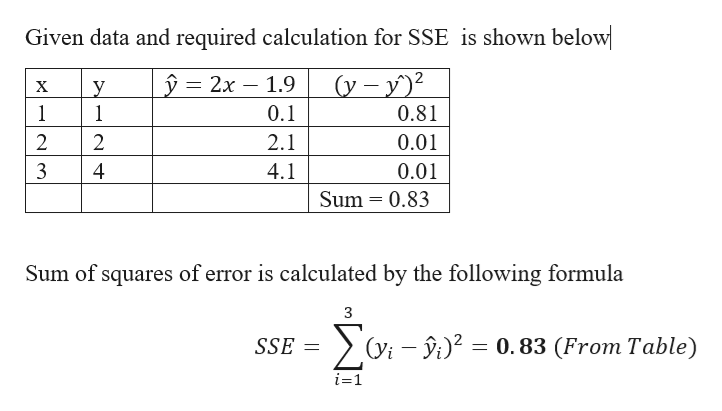# Use technology to compute the sum-of-squares error (SSE) for the given set of data and linear models.(1, 1), (2, 2), (3, 4)(a)    y = 1.5x − 1SSE =  (b)    y = 2x − 1.9SSE =

Question
23 views

Use technology to compute the sum-of-squares error (SSE) for the given set of data and linear models.

(1, 1), (2, 2), (3, 4)
(a)
y = 1.5x − 1

SSE =

(b)
y = 2x − 1.9

SSE =
check_circle

Step 1

Given data and required calculation for SSE  is shown belowhelp_outlineImage Transcriptionclosey = (y-y 1.5x 1 у 1 1 х 0.5 0.25 2 2 2 3 4 3.5 0.25 Sum 0.5 Sum of squares of error is calculated by the following formula 3 (yi 20.5 (From Table) SSE i-1 fullscreen
Step 2

b)

Given data and required calculation ...help_outlineImage TranscriptioncloseGiven data and required calculation for SSE is shown below y = 2x 1.9 (y-y X y 1 0.1 0.81 2 2 2.1 0.01 4 4.1 0.01 Sum 0.83 Sum of squares of error is calculated by the following formula 0.83 (From Table) SSE i=1 fullscreen

### Want to see the full answer?

See Solution

#### Want to see this answer and more?

Solutions are written by subject experts who are available 24/7. Questions are typically answered within 1 hour.*

See Solution
*Response times may vary by subject and question.
Tagged in

### Other